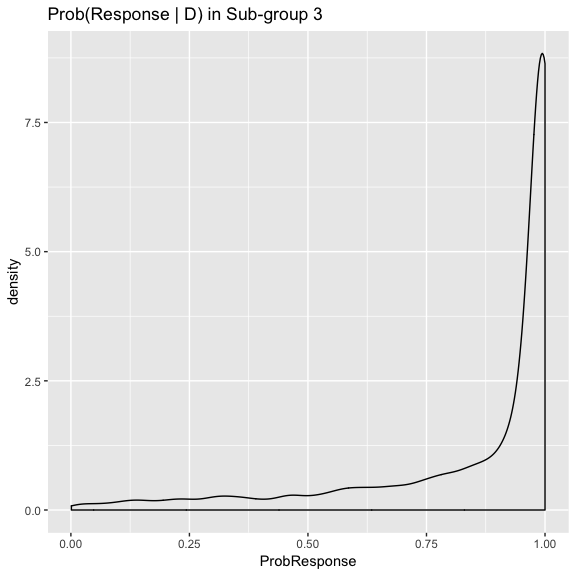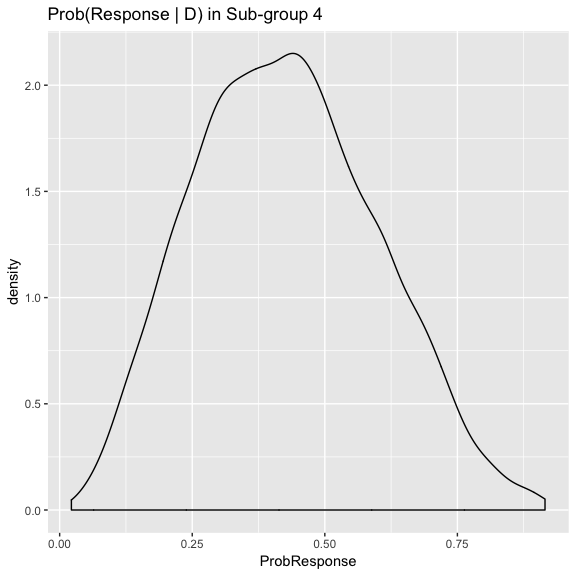# Hierarchical Bayesian model for binary responses

Thall et al. (2003) described a method for analysing treatment effects of a common intervention in several sub-types of a single disease. The treatment effects are assumed to be different but exchangeable and correlated. Observing good efficacy in one cohort, for example, increases one’s expectations of efficacy in other cohorts.

They demonstrate the hierarchical model in a trial with binary response outcomes and in another with time-to-event outcomes. This vignette describes the sarcoma example with binary response outcomes. The authors provide WinBUGS code in the appendix of their paper (Thall et al. 2003). We port their model to Stan and illustrate usage with the example given in their paper.

# Implementation in trialr

The model is compiled in trialr under the ThallHierarchicalBinary slot of the stanmodels object.

Statistically, the authors assume that in a trial of $$k$$ disease subtypes, the treatment effects are drawn from the same common normal distribution

$$\rho_1, ..., \rho_k \sim N(\mu, \sigma^2)$$

As is the convention in BUGS, the authors define normal distributions by a precision parameter $$\tau$$ as opposed to the standard deviation parameter $$\sigma$$ used here. We have re-specified the model to comply with the Stan convention of using standard deviation. The authors use a normal hyperprior on $$\mu$$, and a gamma hyperprior on $$\tau$$, equivalent to an inverse gamma hyperprior on $$\sigma$$.

We observe $$x_i$$ responses in $$n_i$$ patients in disease sub-type $$i$$. The rate of response in subtype $$i$$ is modelled as $$p_i = \text{logit}^{-1}(\rho_i)$$. Each $$x_i$$ is assumed to be binomially distributed with success parameter $$p_i$$. In Stan, that relationship is described as x ~ binomial_logit(n, rho);

The treatment is judged to be worthy of further investigation in cohort $$i$$ if

$$\text{Pr}\left\{ p_i > \theta | \mathcal{D} \right\} > q$$

where $$\theta$$ is the minimum required response rate, and $$q$$ is the required certainty to approve. In their provided example, Thall et al. (2003) use $$\theta = 0.3$$.

# Example

We can learn about the parameters required by the ThallHierarchicalBinary model using

library(trialr)
help(thallhierarchicalbinary_parameters)
## No documentation for 'thallhierarchicalbinary_parameters' in specified packages and libraries:
## you could try '??thallhierarchicalbinary_parameters'

We can get an example using

dat <- thallhierarchicalbinary_parameters_demo()
dat
## $m ##  10 ## ##$x
##   0 0 1 3 5 0 1 2 0 0
##
## $n ##  0 2 1 7 5 0 2 3 1 0 ## ##$target_resp
##  0.3
##
## $mu_mean ##  -1.3863 ## ##$mu_sd
##  3.162278
##
## $tau_alpha ##  2 ## ##$tau_beta
##  20

We have outcomes in 10 disease subgroups. The number of responses is stored in x and the number of patients in n. There have been 3 / 7 responses in subgroup 4, for example, but 0 / 2 responses in subgroup 2, and zero patients treated at all in subgroups 1, 6 and 10.

We invoke the model by calling rstan::sampling on the included ThallHierarchicalBinary model. We set the seed for reproducble randomness.

samp <- rstan::sampling(stanmodels$ThallHierarchicalBinary, data = dat, seed = 123) ## trying deprecated constructor; please alert package maintainer The probability that the efficacy probability in each subgroup exceeds the threshold is stored as pg. We extract that variable and use the rstan::summary function to make inference. knitr::kable(rstan::summary(samp, par = 'pg')$summary, digits = 3)
mean se_mean sd 2.5% 25% 50% 75% 97.5% n_eff Rhat
pg 0.542 0.008 0.498 0 0 1 1 1 3535.715 1.000
pg 0.088 0.004 0.284 0 0 0 0 1 4071.151 0.999
pg 0.946 0.004 0.225 0 1 1 1 1 3393.444 1.000
pg 0.748 0.008 0.434 0 0 1 1 1 2862.118 1.002
pg 1.000 NaN 0.016 1 1 1 1 1 NaN 1.000
pg 0.516 0.008 0.500 0 0 1 1 1 3784.143 1.000
pg 0.708 0.008 0.455 0 0 1 1 1 3087.661 1.001
pg 0.905 0.006 0.294 0 1 1 1 1 2494.959 1.002
pg 0.158 0.006 0.365 0 0 0 0 1 3791.936 1.000
pg 0.544 0.009 0.498 0 0 1 1 1 3320.298 1.000

Let us say that we are willing to approve the treatment for further study in a subgroup if we have at least $$q = 70$$% certainty that the probability of efficacy exceeds the target response rate of 30% On that basis, at this interim stage, we would be willing to approve the treatment in subgroups 3, 4, 5 and 8.

Some distribution plots of the probabilities of efficacy in some subgroups may be informative.

library(ggplot2)
library(rstan)
library(dplyr)

as.data.frame(samp, 'p') %>%
mutate(ProbResponse = p) %>%
ggplot(aes(x = ProbResponse)) +
geom_density() +
ggtitle('Prob(Response | D) in Sub-group 3')Prob(Response | D) in subgroup 3

We see that the inferred efficacy in subgroup 3 is very high.

as.data.frame(samp, 'p') %>%
mutate(ProbResponse = p) %>%
ggplot(aes(x = ProbResponse)) +
geom_density() +
ggtitle('Prob(Response | D) in Sub-group 4')Prob(Response | D) in subgroup 4

In contrast, efficacy is subject to more uncertainty in subgroup 4, but the majority of the mass clearly lies to the right of 30%.

# trialr

trialr is available at https://github.com/brockk/trialr